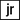# Axis-aligned crate packing

Project originally posted on reddit.com/r/dailyprogrammer

# Description

You have a 2-dimensional rectangular crate of size X by Y, and a bunch of boxes, each of size x by y. The dimensions are all positive integers.

Given X, Y, x, and y, determine how many boxes can fit into a single crate if they have to be placed so that the x-axis of the boxes is aligned with the x-axis of the crate, and the y-axis of the boxes is aligned with the y-axis of the crate. That is, you can’t rotate the boxes. The best you can do is to build a rectangle of boxes as large as possible in each dimension.

For instance, if the crate is size X = 25 by Y = 18, and the boxes are size x = 6 by y = 5, then the answer is 12. You can fit 4 boxes along the x-axis (because 6*4 <= 25), and 3 boxes along the y-axis (because 5*3 <= 18), so in total you can fit 4*3 = 12 boxes in a rectangle.

# Examples

``````fit1(25, 18, 6, 5) => 12
fit1(10, 10, 1, 1) => 100
fit1(12, 34, 5, 6) => 10
fit1(12345, 678910, 1112, 1314) => 5676
fit1(5, 100, 6, 1) => 0``````

## Solution

``````function fit1(X, Y, x, y) {
const sideX = Math.floor(X / x);
const sideY = Math.floor(Y / y);

return sideX * sideY;
}``````